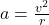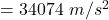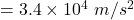## A hard drive disk rotates at 7200 rpm. The disk has a diameter of 5.1 in (13 cm). What is the speed of a point 6.0 cm from the center axle

Question

A hard drive disk rotates at 7200 rpm. The disk has a diameter of 5.1 in (13 cm). What is the speed of a point 6.0 cm from the center axle

in progress 0
5 months 2021-08-17T07:06:21+00:00 1 Answers 1 views 0

The speed will be “3.4×10⁴ m/s²“.

Explanation:

The given values are:

Angular speed,

w = 7200 rpm

i.e.,

=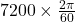=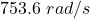Speed from the center,

r = 6.0 cm

As we know,

⇒  Linear speed,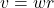On putting the estimated values, we get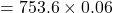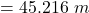Now,

Acceleration on disk will be:

⇒